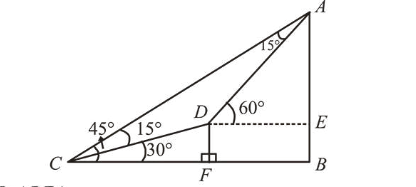# The angle of elevation of the summit of a mountainQuestion:

The angle of elevation of the summit of a mountain from a point on the ground is $45^{\circ}$. After climbing up on $\mathrm{km}$ towards the summit at an inclination of $30^{\circ}$ from the ground, the angle of elevation of the summit is found to be $60^{\circ}$. Then the height (in $\mathrm{km}$ ) of the summit from the ground is:

1. (1) $\frac{\sqrt{3}-1}{\sqrt{3}+1}$

2. (2) $\frac{\sqrt{3}+1}{\sqrt{3}-1}$

3. (3) $\frac{1}{\sqrt{3}-1}$

4. (4) $\frac{1}{\sqrt{3}+1}$

Correct Option: , 3

Solution:

$\because \angle D C A=\angle D A C=30^{\circ}$

$\therefore A D=D C=1 \mathrm{~km}$In $\triangle D E A$,

$\frac{A E}{A D}=\sin 60^{\circ} \Rightarrow A E=\frac{\sqrt{3}}{2} \mathrm{~km}$

In $\Delta C D F, \sin 30^{\circ}=\frac{D F}{C D} \Rightarrow D F=\frac{1}{2} \mathrm{~km}$

$\therefore E B=D F=\frac{1}{2} \mathrm{~km}$

$\therefore$ Height of mountain $=A E+E B$

$=\left(\frac{\sqrt{3}}{2}+\frac{1}{2}\right)=\left(\frac{\sqrt{3}+1}{2}\right) \mathrm{km}=\frac{1}{\sqrt{3}-1} \mathrm{~km}$